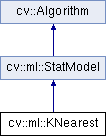OpenCV  3.4.16-dev Open Source Computer Vision
cv::ml::KNearest Class Referenceabstract

The class implements K-Nearest Neighbors model. More...

#include <opencv2/ml.hpp>

Inheritance diagram for cv::ml::KNearest:## Public Types

enum  Types {
BRUTE_FORCE =1,
KDTREE =2
}
Implementations of KNearest algorithm. More...Public Types inherited from cv::ml::StatModel
enum  Flags {
UPDATE_MODEL = 1,
RAW_OUTPUT =1,
COMPRESSED_INPUT =2,
PREPROCESSED_INPUT =4
}

## Public Member Functions

virtual float findNearest (InputArray samples, int k, OutputArray results, OutputArray neighborResponses=noArray(), OutputArray dist=noArray()) const =0
Finds the neighbors and predicts responses for input vectors. More...

virtual int getAlgorithmType () const =0

virtual int getDefaultK () const =0

virtual int getEmax () const =0

virtual bool getIsClassifier () const =0

virtual void setAlgorithmType (int val)=0

virtual void setDefaultK (int val)=0

virtual void setEmax (int val)=0

virtual void setIsClassifier (bool val)=0Public Member Functions inherited from cv::ml::StatModel
virtual float calcError (const Ptr< TrainData > &data, bool test, OutputArray resp) const
Computes error on the training or test dataset. More...

virtual bool empty () const CV_OVERRIDE
Returns true if the Algorithm is empty (e.g. in the very beginning or after unsuccessful read. More...

virtual int getVarCount () const =0
Returns the number of variables in training samples. More...

virtual bool isClassifier () const =0
Returns true if the model is classifier. More...

virtual bool isTrained () const =0
Returns true if the model is trained. More...

virtual float predict (InputArray samples, OutputArray results=noArray(), int flags=0) const =0
Predicts response(s) for the provided sample(s) More...

virtual bool train (const Ptr< TrainData > &trainData, int flags=0)
Trains the statistical model. More...

virtual bool train (InputArray samples, int layout, InputArray responses)
Trains the statistical model. More...Public Member Functions inherited from cv::Algorithm
Algorithm ()

virtual ~Algorithm ()

virtual void clear ()
Clears the algorithm state. More...

virtual String getDefaultName () const

virtual void read (const FileNode &fn)
Reads algorithm parameters from a file storage. More...

virtual void save (const String &filename) const

virtual void write (FileStorage &fs) const
Stores algorithm parameters in a file storage. More...

void write (const Ptr< FileStorage > &fs, const String &name=String()) const
simplified API for language bindings This is an overloaded member function, provided for convenience. It differs from the above function only in what argument(s) it accepts. More...

## Static Public Member Functions

static Ptr< KNearestcreate ()
Creates the empty model. More...

static Ptr< KNearestload (const String &filepath)
Loads and creates a serialized knearest from a file. More...Static Public Member Functions inherited from cv::ml::StatModel
template<typename _Tp >
static Ptr< _Tp > train (const Ptr< TrainData > &data, int flags=0)
Create and train model with default parameters. More...Static Public Member Functions inherited from cv::Algorithm
template<typename _Tp >
static Ptr< _Tp > load (const String &filename, const String &objname=String())
Loads algorithm from the file. More...

template<typename _Tp >
static Ptr< _Tp > loadFromString (const String &strModel, const String &objname=String())
Loads algorithm from a String. More...

template<typename _Tp >
static Ptr< _Tp > read (const FileNode &fn)
Reads algorithm from the file node. More...Protected Member Functions inherited from cv::Algorithm
void writeFormat (FileStorage &fs) const

## Detailed Description

The class implements K-Nearest Neighbors model.

K-Nearest Neighbors

## ◆ Types

Implementations of KNearest algorithm.

Enumerator
BRUTE_FORCE
KDTREE

## ◆ create()

 static Ptr cv::ml::KNearest::create ( )
static
Python:
cv.ml.KNearest_create() -> retval

Creates the empty model.

The static method creates empty KNearest classifier. It should be then trained using StatModel::train method.

## ◆ findNearest()

 virtual float cv::ml::KNearest::findNearest ( InputArray samples, int k, OutputArray results, OutputArray neighborResponses = noArray(), OutputArray dist = noArray() ) const
pure virtual
Python:
cv.ml_KNearest.findNearest(samples, k[, results[, neighborResponses[, dist]]]) -> retval, results, neighborResponses, dist

Finds the neighbors and predicts responses for input vectors.

Parameters
 samples Input samples stored by rows. It is a single-precision floating-point matrix of  * k size. k Number of used nearest neighbors. Should be greater than 1. results Vector with results of prediction (regression or classification) for each input sample. It is a single-precision floating-point vector with  elements. neighborResponses Optional output values for corresponding neighbors. It is a single- precision floating-point matrix of  * k size. dist Optional output distances from the input vectors to the corresponding neighbors. It is a single-precision floating-point matrix of  * k size.

For each input vector (a row of the matrix samples), the method finds the k nearest neighbors. In case of regression, the predicted result is a mean value of the particular vector's neighbor responses. In case of classification, the class is determined by voting.

For each input vector, the neighbors are sorted by their distances to the vector.

In case of C++ interface you can use output pointers to empty matrices and the function will allocate memory itself.

If only a single input vector is passed, all output matrices are optional and the predicted value is returned by the method.

The function is parallelized with the TBB library.

## ◆ getAlgorithmType()

 virtual int cv::ml::KNearest::getAlgorithmType ( ) const
pure virtual
Python:
cv.ml_KNearest.getAlgorithmType() -> retval

Algorithm type, one of KNearest::Types.

setAlgorithmType

## ◆ getDefaultK()

 virtual int cv::ml::KNearest::getDefaultK ( ) const
pure virtual
Python:
cv.ml_KNearest.getDefaultK() -> retval

Default number of neighbors to use in predict method.

setDefaultK

## ◆ getEmax()

 virtual int cv::ml::KNearest::getEmax ( ) const
pure virtual
Python:
cv.ml_KNearest.getEmax() -> retval

Parameter for KDTree implementation.

setEmax

## ◆ getIsClassifier()

 virtual bool cv::ml::KNearest::getIsClassifier ( ) const
pure virtual
Python:
cv.ml_KNearest.getIsClassifier() -> retval

Whether classification or regression model should be trained.

setIsClassifier

 static Ptr cv::ml::KNearest::load ( const String & filepath )
static
Python:

Loads and creates a serialized knearest from a file.

Use KNearest::save to serialize and store an KNearest to disk. Load the KNearest from this file again, by calling this function with the path to the file.

Parameters
 filepath path to serialized KNearest

## ◆ setAlgorithmType()

 virtual void cv::ml::KNearest::setAlgorithmType ( int val )
pure virtual
Python:
cv.ml_KNearest.setAlgorithmType(val) -> None

## ◆ setDefaultK()

 virtual void cv::ml::KNearest::setDefaultK ( int val )
pure virtual
Python:
cv.ml_KNearest.setDefaultK(val) -> None

getDefaultK

## ◆ setEmax()

 virtual void cv::ml::KNearest::setEmax ( int val )
pure virtual
Python:
cv.ml_KNearest.setEmax(val) -> None